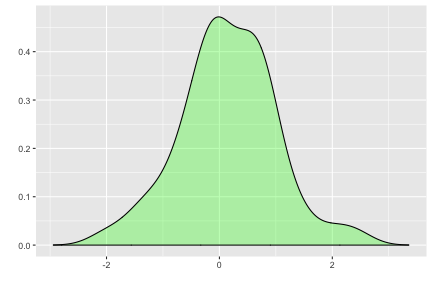This document explains how to plot probability distributions using {ggplot2} and {ggfortify}.

Plotting Probability Distributions

ggdistribution is a helper function to plot Distributions in the stats package easier using ggplot2.

For example, plot standard normal distribution from -3 to +3:

library(ggfortify)
ggdistribution(dnorm, seq(-3, 3, 0.1), mean = 0, sd = 1)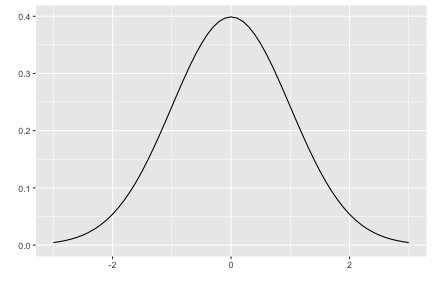ggdistribution accepts PDF/CDF function, sequence, and options passed to PDF/CDF function. Also, it has some options to configure how plot looks. Use help(ggdistribution) to check available options.

ggdistribution(pnorm, seq(-3, 3, 0.1), mean = 0, sd = 1, colour = 'red')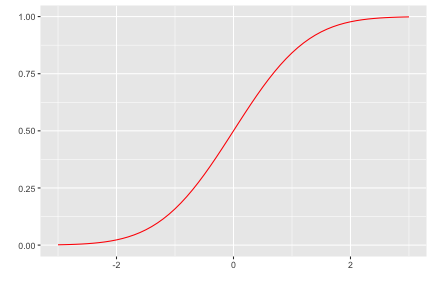ggdistribution(dpois, seq(0, 20), lambda = 9, fill = 'blue')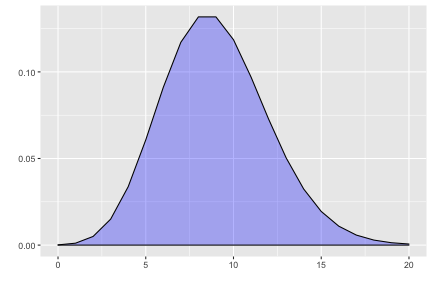If you want to plot some distributions overwrapped, use p keyword to pass ggplot instance.

p <- ggdistribution(dchisq, seq(0, 20, 0.1), df = 7, colour = 'blue')
p <- ggdistribution(dchisq, seq(0, 20, 0.1), df = 9, colour = 'green', p = p)
ggdistribution(dchisq, seq(0, 20, 0.1), df = 11, colour = 'red', p = p)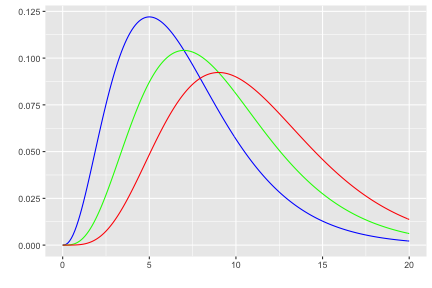Plotting Density

Also, autoplot can accept stats::density.

autoplot(density(rnorm(1:50)), fill = 'green')